# Suggested Model for Heat Transfer Calculation During Fluid Flow in Single Phase Inside Pipes (II)

Suggested Model for Heat Transfer Calculation During Fluid Flow in Single Phase Inside Pipes (II)

Yanán Camaraza-MedinaKen Mortensen-Carlson Pratijay Guha Ángel M. Rubio-Gonzales Oscar M. Cruz-Fonticiella Osvaldo F. García-Morales

Center of Energy Studies and Environmental Technology, Universidad Central “Marta Abreu” de Las Villas 54440, Cuba

Department of Chemical Engineering, University of California, Santa Bárbara CA 93106, USA

Department of Mechanical Engineering, Birla Institute of Technology and Sciences, Pilani Hyderabad 333031, India

Technical Sciences Faculty, Universidad de Matanzas, Matanzas 44440, Cuba

Corresponding Author Email:
ycamaraza1980@yahoo.com
Page:
257-266
|
DOI:
https://doi.org/10.18280/ijht.370131
11 December 2018
|
Accepted:
5 March 2019
|
Published:
31 March 2019
| Citation

OPEN ACCESS

Abstract:

In this paper, is presented a mathematical deduction of a new improved model for heat transfer calculations during fluid flow in single-phase inside tubes. The proposal model was verified by comparison with available experimental data of 35 different fluids, including water, air, gases and organic substances. The proposal model is valid for a range of Reynolds number for single-phase from 2.4·10to 8.2·106, Prandtl number for single-phase from 0.65 to 4.71·104, dimensionless length in the interval 2≤ld450 and values of Petukhov’s correction in the interval 0006≤μFμP≤177. In 3096 data analyzed, for Re<1·104, the mean deviation found was 13.91% in the 80.32% of the experimental data, while for 1·104Re, the mean deviation found was 13.96% in 80.94% of experimental data.

Keywords:

single phase, model, heat transfer coefficient , average deviation

1. Instruction

Currently, heat transfer calculations for turbulent fluid flow within straight conduits in single-phase media are made by the Dittus-Boelter equation, or by the improved version of Sieder-Tate . This procedure is a requirement for the evaluation of industrial facilities or production equipment. A drawback of these equations is their high dispersion value, reaching compute errors close to ± 40 %.

At the Moscow Energy Institute, Petukhov and his collaborators constructed a model based on experimental quantity adjustments, using the Prandtl analogy as an adjustment function . This equation gives results with a lower margin of error, and allows us to estimate the mean error by the dimensionless number of Prandtl.

if Pr≤200    Error<5%

if Pr≥200    Error≤10%

Although the application of the Petukhov’s Equation is more laborious, the results obtained have a minor dispersion, therefore, a smaller safety margin in the design calculations.

A major drawback is its applicability range, because this is only valid for a fully developed turbulent flow regime, 1∙$10^4$<Re, and is not valid for the flow that operate in the transition zone 2.3∙$10^3$<Re<1∙$10^4$. This problem was later solved by Gnielinsky [3-4], who modified the Petukhov’s Equation, adjusting it to experimental data that do take into account the transition flow zone.

In the literature can be found an important group of works that facilitate the calculation of heat transfer inside of straight tubes with turbulent flow, this is mainly associated with the changing nature of the turbulent flow, which hinders the development of analytical expressions. This element makes it necessary to resort to the experimentation and subsequent adjustment of experimental quantities through the theory of dimensional analysis.

2. Methods and Validation

2.1 Analogy between heat transfer and momentum in single-phase fluid flow inside pipes

The Darcy friction factor f allows determining the heat transfer coefficient α, by analogy between heat transfer and momentum. The shear stress $\tau$ in the turbulent boundary layer is composed of two terms :

$\tau ={{\tau }_{Visc}}+{{\tau }_{Turb}}=\mu \frac{dV}{dx}-\rho V_{X}^{*}V_{Y}^{*}$ (1)

In Equation (1) ${\tau }_{Turb}$ is the Reynolds stress; $V*_X$ is the fluctuation of the instantaneous velocity $V^M_X$ in the coordinate axis x; $V*_Y$ is the fluctuation of the instantaneous velocity $V^M_X$ in the coordinate axis y.

The instantaneous velocity $V^M_X$ and $V^M_Y$ are determined as:

$V_{X}^{M}=V_{M}^{X}\pm V_{X}^{*}=V_{M}^{X}\pm V_{agit}^{X}$  (2)

$V_{Y}^{M}=V_{M}^{Y}\pm V_{Y}^{*}=V_{M}^{Y}\pm V_{agit}^{Y}$   (3)

For turbulent heat flow, it can be considered that the total heat flow $q*$ is composed of a sum that includes the conductive component $q_{cond}$ and the turbulent component $q_{turb}$, then:

${{q}^{*}}={{q}_{cond}}+{{q}_{turb}}=-\lambda \frac{dT}{dx}+\rho CpV_{Y}^{*}T_{F}^{*}$ (4)

In Equation (3) there are three temperature references, which are:

\left\{ \begin{align} & T_{F}^{*}={{T}_{F}}\pm T_{F}^{*}\ \text{,}\ \text{ }\quad \quad \to \text{is the instantaneous temperature} \\ & {{T}_{F}}={{T}_{\infty }}\ \text{, }\ \quad \quad \quad \quad \to \text{is the average temperature of the fluid} \\ & T_{F}^{*}\ \text{, }\ \quad \quad \quad \quad \quad \quad \to \text{is the temperature due to the fluctuation } \\ \end{align} \right.  (5)

Terms $V*_X$ and $V*_Y$ are obtained from their physical meaning from the Prandtl mixing number, which suggests that the fluctuation of velocity $V*_X$ is related with $dV/dx$ as:

$V_{X}^{*}\approx {{L}_{M}}\frac{dV}{dx}$ (6)

In Equation (6), $L_M$ is the mixture length of the thickness film ${{\delta }_{2}}$ of the momentum in boundary layer. Similarly, transverse fluctuation $V*_Y$ is admitted to be of the same order of magnitude $V*_X$ but opposite in sign, :

$V_{Y}^{*}\approx -{{L}_{M}}\frac{dV}{dx}$ (6.a)

Combining the Equations (6) and (6.a):

$V_{X}^{*}V_{Y}^{*}\approx -{{\left( {{L}_{M}}\frac{dV}{dx} \right)}^{2}}$  (7)

Equation (7) can be transformed to:

$V_{X}^{*}V_{Y}^{*}\approx {{\varepsilon }_{M}}{dV}/{dx}\;$  (8)

In Equation (8) ${{\varepsilon }_{M}}$ is the momentum turbulent diffusivity, then:

${{\varepsilon }_{M}}\approx L_{M}^{2}{dV}/{dx}\;$ (9)

To find the relationship of the term $V_{Y}^{*}T_{F}^{*}$, with the mean local temperature gradient, a similar method is applied, in the form .

$T_{F}^{*}=-{{L}_{C}}\frac{dT}{dx}$ (10)

$V_{Y}^{*}={{L}_{C}}{dV}/{dx}\;$ (11)

In the expression (11) $L_C$ is the mixture length of the energy in the thickness ${{\delta }_{3}}$ of the thermal boundary layer, then:

$V_{Y}^{*}T_{F}^{*}=-L_{C}^{2}\frac{dV}{dx}\frac{dT}{dx}=-{{\varepsilon }_{C}}\frac{dT}{dx}$ (12)

In the Equation (12), the term ${{\varepsilon }_{C}}=-L_{C}^{2}{dV}/{dx}\;$ is the heat turbulent diffusivity. Substituting Equation (8) into Equation (1):

$\tau ={{\tau }_{Visc}}+{{\tau }_{Turb}}=\mu {dV}/{dx}\;-\rho {{\varepsilon }_{M}}{dV}/{dx}\;$  (13)

Dividing by the density $\rho$ to both members of the Equation (13) and taken the derivative ${dV}/{dx}\;$ as a common factor [7-8], then:

$\frac{\tau }{\rho }={{\tau }_{Visc}}+{{\tau }_{Turb}}=\left( \frac{\mu }{\rho }+{{\varepsilon }_{M}} \right)\frac{dV}{dx}=\left( \nu +{{\varepsilon }_{M}} \right)\frac{dV}{dx}$ (14)

Substituting Equation (12) into Equation (4):

${{q}^{*}}={{q}_{cond}}+{{q}_{turb}}=-\lambda \frac{dT}{dx}-\rho Cp{{\varepsilon }_{C}}\frac{dT}{dx}$ (15)

If in Equation (15) the derivative ${dT}/{dx}\;$ is taken as a common factor, then

${{q}^{*}}={{q}_{cond}}+{{q}_{turb}}=-\left( \lambda +\rho Cp{{\varepsilon }_{C}} \right){dT}/{dx}\;$ (16)

In Equation (16), both members are divided by the product of the density and specific heat at constant pressure $\rho Cp$.

$\frac{{{q}^{*}}}{\rho Cp}=-\left( \frac{\lambda }{\rho Cp}+{{\varepsilon }_{C}} \right)\frac{dT}{dx}=-\left( a+{{\varepsilon }_{C}} \right)\frac{dT}{dx}$ (17)

Dividing Equation (17) by the Equation (14) is obtained the basic relationships for the fluid flow inside of tubes [6-9]:

$\frac{\tau }{{{q}^{*}}}=-\frac{\nu +{{\varepsilon }_{M}}}{Cp\left( a+{{\varepsilon }_{C}} \right)}\frac{dV}{dT}$  (18)

In Equation (18), the kinematic viscosity v and the thermal diffusivity a are properties of the fluid, while ${{\varepsilon }_{C}}$ and ${{\varepsilon }_{M}}$ are properties of the flow.

2.2 Development of one linear model for convective heat transfer calculation in single-phase inside pipes

Development of the new model for to calculate the convective heat transfer in single-phase inside pipes is a complex task. Initially is taken the criterion established by Prandtl, which considers that the flow is divided into two zones, a viscous zone and a turbulent zone. In his analysis Prandtl makes the additional assumptions that in the turbulent zone the molecular diffusivities of momentum v and of heat a, are negligible in comparison with the turbulent diffusivities $\nu <<{{\varepsilon }_{M}}$; $a<<{{\varepsilon }_{C}}$, so they do not intervene in the process. It would be very useful for such a purpose to assume that the relationship between molecular diffusivities a and v is equal to the relationship between diffusivities ${{\varepsilon }_{C}}$ and ${{\varepsilon }_{M}}$.

Since the dimensionless Prandtl number is a relation between diffusivities, then the previous assumption is fulfilled .

$\Pr =\frac{\nu }{a}=\frac{{{\varepsilon }_{M}}}{{{\varepsilon }_{C}}}$  (19)

Clearing v and ${{\varepsilon }_{M}}$ in the Equation (19):

$\nu =a\Pr$ (20)

${{\varepsilon }_{M}}={{\varepsilon }_{C}}\Pr$(21)

Substituting Equations (20) and (21) into Equation (18):

$\frac{\tau }{{{q}^{*}}}=-\frac{a\Pr +{{\varepsilon }_{C}}\Pr }{Cp\left( a+{{\varepsilon }_{C}} \right)}\frac{dV}{dT}$   (22)

If the Prandtl number Pr is taken as a common factor in Equation (22), it is reduced to :

$\frac{\tau }{{{q}^{*}}}=\frac{{{\tau }_{0}}}{q_{0}^{*}}=-\frac{\left( a+{{\varepsilon }_{C}} \right)\Pr }{\left( a+{{\varepsilon }_{C}} \right)Cp}\frac{dV}{dT}=-\frac{\Pr }{Cp}\frac{dV}{dT}$   (23)

Separating variables in (23) and integrating

$\int\limits_{0}^{{{V}_{M}}}{dV}=-\frac{Cp{{\tau }_{0}}}{\Pr q_{0}^{*}}\int\limits_{{{T}_{P}}}^{{{T}_{F}}}{dT}$  (24)

Resolving the integrals present in Equation (24) and grouping conveniently:

${{V}_{M}}=\frac{Cp{{\tau }_{0}}}{\Pr q_{0}^{*}}\left( {{T}_{P}}-{{T}_{F}} \right)$   (25)

In Equation (25) the terms ${{\tau }_{0}}$ and $q_{0}^{*}$ are taken on the surface. It is known from the fluid mechanics courses that :

$\pi dL{{\tau }_{0}}=\Delta p\frac{\pi {{d}^{2}}}{4}$  (26)

Clearing $\Delta p$ in Equation (26)

$\Delta p={4L{{\tau }_{0}}}/{d}\;$ (27)

The Darcy Equation for surface is:

$\Delta p=f\frac{l}{d}\frac{{{V}^{2}}}{2}\rho$  (28)

Equaling the Equations (28) and (27)

$\frac{4L{{\tau }_{0}}}{d}=f\frac{L\rho V_{M}^{2}}{2d}$ (29)

The shear stress ${{\tau }_{0}}$ on the surface is given in the left term of the Equation (29), therefore, clearing it, can be obtaining the expression that allows determining the shear stress ${{\tau }_{0}}$ on the surface .

${{\tau }_{0}}={f\rho V_{M}^{2}}/{8}\;$  (30)

The mean drag coefficient is taken as a quarter of the Darcy friction factor

${{C}_{W}}={f}/{4}\;$ (31)

Then, substituting the Equation (31) into Equation (30)

${{\tau }_{0}}=\frac{{{C}_{W}}\rho V_{M}^{2}}{2}$ (32)

The quantity of heat transferred is obtained with the Newton’s law of cooling [11-13].

$q_{0}^{*}=\alpha \left( {{T}_{P}}-{{T}_{F}} \right)$ (33)

Substituting the Equations (33) and (32) into Equation (25):

${{V}_{M}}=\frac{Cp\,f\,\rho \,V_{M}^{2}}{8\Pr \alpha \left( {{T}_{P}}-{{T}_{F}} \right)}\left( {{T}_{P}}-{{T}_{F}} \right)$ (34)

Equation (34) is transformed to:

${{V}_{M}}=\frac{Cp\,f\,\rho \,V_{M}^{2}}{8\Pr \alpha }$ (35)

Clearing the mean heat transfer coefficient in Equation (35):

$\alpha =\frac{Cp\,f\,\rho \,{{V}_{M}}}{8\Pr }$ (36)

Equation (36) contains all the physical properties necessary to form the Stanton dimensionless group.

$St=\frac{Nu}{\operatorname{Re}\Pr }=\frac{\alpha }{\rho CpV}$  (37)

Substituting Equation (37) into Equation (36)

$\frac{\alpha }{Cp\rho {{V}_{M}}}=St=\frac{Nu}{\operatorname{Re}\Pr }=\frac{f}{8\Pr }$  (38)

From the Equation (31), Equation (38) is transformed to:

$\frac{\alpha }{Cp\rho {{V}_{M}}}=St=\frac{Nu}{\operatorname{Re}\Pr }=\frac{{{C}_{W}}}{2\Pr }$  (39)

Solving the average drag coefficient ${{C}_{W}}$ in the Equation (39)

$\frac{\alpha}{CpρV_M}=\frac{Nu}{RePr}=St=\frac{C_W}{2Pr}$ (39.a)

Equation (39.a) is a good approximation to the model given by Pohlhausen [12-13].

${{C}_{W}}=2St\Pr$ (40)

Equation (40) agrees very well with the experimental values for $\Pr \approx 1$. The friction factor is obtained with the Equation given by Eckert [12-14].

$f=0,184{{\operatorname{Re}}^{-0,2}}$ (41)

Equation (41) is valid for:

${{10}^{4}}<\operatorname{Re}<{{10}^{5}}\quad \quad ;\quad \quad \frac{L}{d}=0,623\sqrt{\operatorname{Re}}$ (42)

In Equation (42) L is the initial section of hydrodynamic compensation (necessary distance so that in turbulent flow the Darcy's f friction factor becomes constant). Substituting the Equations (41) into Equation (40):

\begin{align} & Nu=St\operatorname{Re}\Pr =\frac{f}{8\sqrt{\Pr }}\operatorname{Re}\Pr = \\ & =\frac{0,184}{8}{{\operatorname{Re}}^{0,8}}{{\Pr }^{{1}/{3}\;}}=0,023{{\operatorname{Re}}^{0,8}}{{\Pr }^{{1}/{3}\;}} \\ \end{align}  (43)

Equation (43) is valid for:

${{10}^{4}}<\operatorname{Re}<{{10}^{5}}\quad ;\quad \frac{L}{d}>60\quad ;\quad 0,5<\Pr <100$ (44)

Equation (43) was later modified by Dittus-Boelter , where, the exponent 1/3 from the Prandtl number was substituted by the constant n, which takes values of 0.3 and 0.4 for cooling and heating respectively. This action broadens the area of applicability of Equation (43) to [16-18]:

${{10}^{4}}<\operatorname{Re}\quad ;\quad \frac{L}{d}>60\quad ;\quad 0,5<\Pr <160$  (45)

2.3 Deduction and development of the proposal model

If it is considered that ${\nu }/{a}\;=1$, then ${{\varepsilon }_{M}}={{\varepsilon }_{C}}$ and the Equation (23) is transformed to:

$\frac{\tau }{{{q}^{*}}}=\frac{{{\tau }_{0}}}{q_{0}^{*}}=-\frac{{{\varepsilon }_{M}}}{{{\varepsilon }_{C}}}\frac{dV}{CpdT}=-\frac{dV}{CpdT}$ (46)

In the viscous sublayer it is satisfied that $\nu >>{{\varepsilon }_{M}}$ $a>>{{\varepsilon }_{C}}$, then, transforming the Equation (46):

$\frac{\tau }{{{q}^{*}}}=\frac{{{\tau }_{0}}}{q_{0}^{*}}=-\frac{\nu }{a}\frac{dV}{CpdT}=-\frac{\Pr }{Cp}\frac{dV}{dT}$ (47)

Separating variables in Equation (47), assuming that the profile of temperature distribution on the turbulent boundary layer is approximately a parabolic-exponential curve, we obtain:

$dT=-{{\Pr }^{{2}/{3}\;}}\frac{q_{0}^{*}}{Cp{{\tau }_{0}}}dV$  (48)

Integrating in the Equation (48), the left member between the wall temperatures $T_p$ and the temperature on the edge of the viscous boundary layer $T_1$, the member on the right is integrated in the interval from zero until the edge velocity in the tube wall.

$\int\limits_{{{T}_{P}}}^{{{T}_{1}}}{dT}=\int\limits_{0}^{{{V}_{1}}}{-{{\Pr }^{{2}/{3}\;}}\frac{q_{0}^{*}}{Cp{{\tau }_{0}}}dV}$  (49)

Solving the integrals present in Equation (49):

${{T}_{P}}-{{T}_{1}}=-{{\Pr }^{{2}/{3}\;}}\frac{q_{0}^{*}}{Cp{{\tau }_{0}}}{{V}_{1}}$  (50)

Separating variables in the Equation (46):

$dT=-\frac{q_{0}^{*}}{Cp{{\tau }_{0}}}dV$ (51)

Integrating the Equation (51), in the left member, between the temperature on the edge of the viscous boundary layer and the average temperature of the fluid flow. The right member is integrated in the interval between the velocity on the edge of the viscous boundary layer and the mean velocity of the fluid stream, then:

$\int\limits_{{{T}_{1}}}^{{{T}_{F}}}{dT}=\int\limits_{{{V}_{1}}}^{{{V}_{M}}}{-P\frac{q_{0}^{*}}{Cp{{\tau }_{0}}}dV}$  (52)

Resolving the integrals present in Equation (52)

${{T}_{1}}-{{T}_{F}}=\frac{q_{0}^{*}}{Cp{{\tau }_{0}}}\left( {{V}_{M}}-{{V}_{1}} \right)$  (53)

Adding the Equations (53) and (50), we obtain :

\begin{align} & {{T}_{P}}-{{T}_{1}}+{{T}_{1}}-{{T}_{F}}=-{{\Pr }^{{2}/{3}\;}}\frac{q_{0}^{*}}{Cp{{\tau }_{0}}}{{V}_{1}}+ \\ & +\frac{q_{0}^{*}}{Cp{{\tau }_{0}}}\left( {{V}_{M}}-{{V}_{1}} \right) \\ \end{align}   (54)

Grouping terms in Equation (54) is up to:

${{T}_{P}}-{{T}_{F}}=\frac{q_{0}^{*}{{V}_{M}}}{Cp{{\tau }_{0}}}\left[ 1+\frac{{{V}_{1}}}{{{V}_{M}}}\left( {{\Pr }^{{2}/{3}\;}}-1 \right) \right]$ (55)

Substituting Equations (30) and (33) into Equation (55)

${{T}_{P}}-{{T}_{F}}=\frac{8\alpha \left( {{T}_{P}}-{{T}_{F}} \right){{V}_{M}}}{Cp\,f\,\rho \,V_{M}^{2}}\left[ 1+\frac{{{V}_{1}}}{{{V}_{M}}}\left( {{\Pr }^{{2}/{3}\;}}-1 \right) \right]$(56)

Clearing the mean heat transfer coefficient $\alpha$ in the Equation (56) and grouping conveniently is finally obtained [20-21]:

$\alpha =\frac{f}{8}\frac{\rho Cp{{V}_{M}}}{\left[ 1+\frac{{{V}_{1}}}{{{V}_{M}}}\left( {{\Pr }^{{2}/{3}\;}}-1 \right) \right]}$  (57)

Substituting Equation (37) into Equation (57)

$\frac{\alpha }{Cp\rho {{V}_{M}}}=St=\frac{Nu}{\operatorname{Re}\Pr }=\frac{f}{8\left[ 1+\frac{{{V}_{1}}}{{{V}_{M}}}\left( {{\Pr }^{{2}/{3}\;}}-1 \right) \right]}$  (58)

The velocity on the edge of the viscous boundary layer V1 is determined with the aid of the law of velocities distribution for turbulent flows, applying the Schlichting Equation :

$\frac{{{\tau }_{0}}}{\rho }={{\left( \frac{{{V}_{1}}}{12,7} \right)}^{2}}=\frac{fV_{M}^{2}}{8}$ (59)

Clearing the velocities of the left member in Equation (59), we obtain that:

$\frac{{{V}_{1}}}{{{V}_{M}}}=12,7\sqrt{\frac{f}{8}}$   (60)

Substituting Equation (60) into Equation (58) gives the final Stanton number.

$\frac{\alpha }{Cp\rho {{V}_{M}}}=St=\frac{Nu}{\operatorname{Re}\Pr }=\frac{\frac{f}{8}}{1+12,7\sqrt{\frac{f}{8}}\left( {{\Pr }^{{2}/{3}\;}}-1 \right)}$  (61)

or:

$Nu=\frac{f\operatorname{Re}\Pr }{8+\sqrt{1290,3f}\cdot \left( {{\Pr }^{{2}/{3}\;}}-1 \right)}$  (62)

Equation (62) is the starting point for the development of a new model that allows to obtain the coefficient of heat transfer in single phase. This includes a smaller margin of error with respect to the existing models and with a greater range of applicability.

To consider the effect of the variation of the fluid physical properties along of the tube, the Equation (62) is affected by the factor of correction given by Petukhov [16-18]:

$Nu=\frac{f\operatorname{Re}\Pr }{8+\sqrt{1290,3f}\cdot \left( {{\Pr }^{{2}/{3}\;}}-1 \right)}\cdot {{\left( \frac{{{\mu }_{F}}}{{{\mu }_{P}}} \right)}^{N}}$  (63)

In Equation (63), the coefficient N take values 0.25 y 0.11 for cooling and heating of the fluid respectively.

When an initial section of hydrodynamic compensation is not available, it is necessary to include this correction, transforming Equation (63)

\begin{align} & Nu= \\ & =\frac{f\operatorname{Re}\Pr }{8+\sqrt{1290,3f}\cdot \left( {{\Pr }^{{2}/{3}\;}}-1 \right)}\cdot \left( 1+{{\left( \frac{d}{l} \right)}^{{2}/{3}\;}} \right)\cdot {{\left( \frac{{{\mu }_{F}}}{{{\mu }_{P}}} \right)}^{N}} \\ \end{align} (64)

The friction factor is obtained with the application of the Equation of Filonenko [15-16]:

$Nu=\frac{f\operatorname{Re}\Pr }{8+\sqrt{200f}\cdot \left( \Pr -1 \right)}\cdot \left( 1+{{\left( \frac{d}{l} \right)}^{{2}/{3}\;}} \right)\cdot {{\left( \frac{{{\mu }_{F}}}{{{\mu }_{P}}} \right)}^{N}}$  (65)

Equation (65) is conveniently transformed to:

${{f}^{2}}={{\left( \log {{\left( \operatorname{Re} \right)}^{1,82}}-1,64 \right)}^{-1}}$   (66)

Applying logarithm properties in the Equation (66), taking one constant equal to 3.25 as a common factor:

${{f}^{2}}={{\left( 3,25\cdot \left( \log {{\left( \operatorname{Re} \right)}^{1{,82}/{3,25}\;}}-{1,64}/{3,25}\; \right) \right)}^{-1}}$ (67)

Then:

${{f}^{2}}={{\left( 3,25\cdot \left( \log {{\left( \operatorname{Re} \right)}^{0,56}}-0,505 \right) \right)}^{-1}}$ (68)

Simplifying the Equation (68).

${{f}^{2}}={{\left[ 3,25\cdot \log \left( \frac{{{\operatorname{Re}}^{0,56}}}{3,196} \right) \right]}^{-1}}$ (69)

or

$f=\frac{{{\left[ \log \left( \frac{{{\operatorname{Re}}^{0,56}}}{3,196} \right) \right]}^{-2}}}{10,56}\quad \to \quad B=\log \left( \frac{{{\operatorname{Re}}^{0,56}}}{3,196} \right)$ (70)

Then Equation (70) is transformed to:

$f=\frac{{{B}^{-2}}}{10,56}$   (71)

Substituting Equation (71) into Equation (64)

\begin{align} & Nu=\frac{\operatorname{Re}\Pr }{10,56{{B}^{2}}\cdot \left( 8+\sqrt{1290,3\cdot 10,56\cdot {{B}^{2}}}\cdot \left( {{\Pr }^{{2}/{3}\;}}-1 \right) \right)}\cdot \\ & \cdot \left( 1+{{\left( \frac{d}{l} \right)}^{{2}/{3}\;}} \right)\cdot {{\left( \frac{{{\mu }_{F}}}{{{\mu }_{P}}} \right)}^{N}} \\ \end{align} (72)

or

\begin{align} & Nu=\frac{\operatorname{Re}\Pr }{84,5{{B}^{2}}+116,74B\cdot \left( {{\Pr }^{{2}/{3}\;}}-1 \right)}\cdot \\ & \cdot \left( 1+{{\left( \frac{d}{l} \right)}^{{2}/{3}\;}} \right)\cdot {{\left( \frac{{{\mu }_{F}}}{{{\mu }_{P}}} \right)}^{N}} \\ \end{align} (73)

Equation (73) can be written as :

\begin{align} & Nu=\frac{\operatorname{Re}\Pr }{84,5{{B}^{2}}-116,74B\cdot \left( 1-{{\Pr }^{{2}/{3}\;}} \right)}\cdot \\ & \cdot \left( 1+{{\left( \frac{d}{l} \right)}^{{2}/{3}\;}} \right)\cdot {{\left( \frac{{{\mu }_{F}}}{{{\mu }_{P}}} \right)}^{N}} \\ \end{align} (74)

In Equation (74), $A=84.5$ and $D=116.74$

3. Experimental Validation of the Proposed Model

Equation (74) was developed for turbulent flow in single-phase inside pipes. For the transitional zone, in this work, the authors prefer the adjustments obtained with the Gnielinsky's correction, predetermining it as a functional logarithmic of base 10.

$Re\approx(Re-10^D)$  (75)

Applying the Brezhneztov's method, can be obtained the coefficient D as one polynomial curve of second order, dependent of the functional $log(D)$ .

$D=-0.027\cdot {{\left[ \log \left( \operatorname{Re} \right) \right]}^{\,2}}+0,2\log \left( \operatorname{Re} \right)+2,63$ (76)

Figure 1 shows the correlation between the Equation (76) and the experimental data . For transitional zone, constants A and C in Equation (74) are determined through adjustment of experimental data. This correlation is showed in the figures 2 and 3 respectively.

For the turbulent flow regime, the constant D is deleted, while the constants A and C in Equation (74) are determined through adjustment of experimental data . This correlation is showed in the figures 4 and 5 respectively. Table 1 provides a detailed description of the new proposal model for transition and turbulent flow regime.

The proposal model covers a greater range of validity. To show its effectiveness, a correlation is made of the values obtained from the use of Equation (74) and the experimental data available [22-23], dividing the range of applicability into seven subintervals of validity and then the average error rate is determined. The results obtained are determined by determining the percent of average error. The results obtained are summarized in Tables 4 and 5.

## figure_1.png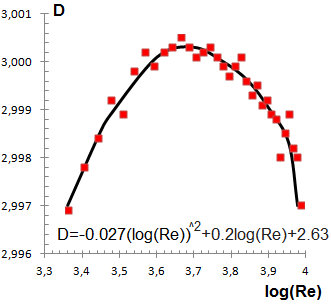Figure 1. Comparison of experimental data with the Equation (76)

## figure_2.png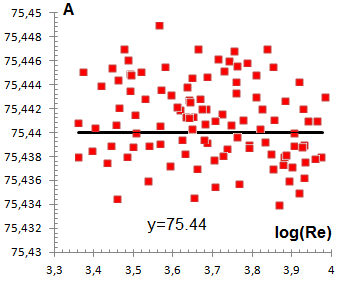Figure 2. Determination of the constant A for the Equation (74) in transition zone

## figure_3.png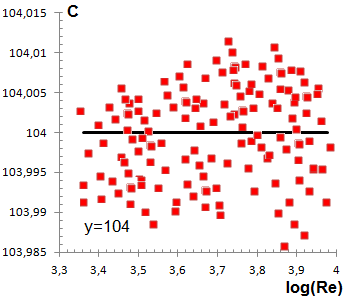Figure 3. Determination of the constant C for the Equation (74) in the transition zone

In the table 4, for the validity range 2.4·$10^3$≤Re≤$10^4$ and 0.65<Pr≤4.71∙$10^4$, the proposal model correlates with an average error of 13.91%, in 80.32% of the available experimental data, then, the obtained adjustment is considered excellent, very similar to those obtained by using the Gnielinsky Equation, which should be clarified that it cannot be used for Pr>2000. It is also observed that for values of Pr<200, the average error obtained is 6.96% for 90.42% of the available data, which brings it numerically to the 5% reported by Gnielinsky.

## figure_4.png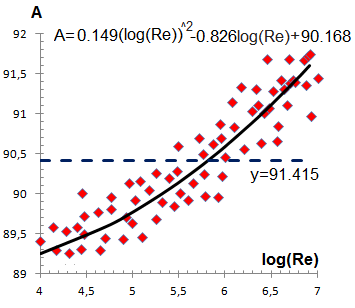Figure 4. Determination of the constant A for the Equation (74) in turbulent flow regime

## figure_5.png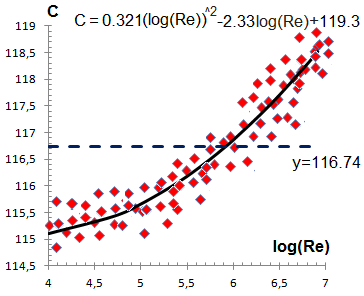Figure 5. Determination of the constant C for the Equation (74) in turbulent flow regime

Table 1. Description of the new proposal model for transition and turbulent flow regime

 Equation (74) \begin{align} & Nu=\frac{(Re-10^D)Pr}{A\cdot {{B}^{2}}-C\cdot B\cdot \left( 1-{{\Pr }^{{2}/{3}\;}} \right)}\cdot \\ & \cdot \left( 1+{{\left( \frac{d}{l} \right)}^{{2}/{3}\;}} \right)\cdot {{\left( \frac{{{\mu }_{F}}}{{{\mu }_{P}}} \right)}^{N}} \\ \end{align} Transition zone    $2.3\cdot 10^3$

In the Table 5 for $10^4≤Re≤8.2\cdot 10^6$ and $0.65<Pr≤4.71\cdot10^4$, the Equation (74) correlates with an average error of 13.96%, in the 80.94% of the available experimental data, so the adjustment obtained is considered to be excellent, very similar to those obtained by using the Equations of Petukhov and Gnielinsky, which should be clarified that it cannot be used for Pr> 2000. It is also observed that for values of Pr <200, the average error obtained is 7.12 % for 88.35% of the available data, which brings it numerically to the 5% reported by Petukhov and Gnielinsky.

Table 2 provides a detailed summary of the range that shows a satisfactory fit with the correlation proposed in the present work.

Table 2. Summary of the validity range for the Equation (74)

 Parameter Range Fluids Water, Air, Helium, Hydrogen, Nitrogen, Carbon Dioxide, Transformer oil, Glycerin, MC Oil, MK Oil, Butyl alcohol, Methanol, Ethanol, Ethylene glycol, Kerosene, Acetic Acid, Acetaldehyde, Butanol, Aniline, Carbon Disulfide, Ciclohexane, Ethyl ether, Ethylamine, Oil olive, Toluene, Turpentine, Propylene, Pentane, Benzene, Gasoline, Isobutene, Engine oil. Decane and Dodecane $Pr$ 0.65 to $4.71\cdot 10^4$ $Re$ $2.4\cdot 10^3$  to  $8.2\cdot 10^6$ $\mu_F/\mu_p$ $0.006≤\mu_F/\mu_p≤177$ $l/d$ $2≤l/d≤420$

In this work, the experimental data used in the validation of the developed model were extracted of the critical review available in the reference , which provides one large data base of experimental data compiled on heat transfer calculation during fluid flow in single-phase inside tubes. Table 3 provides the available experimental data used in this paper.

Table 3. Experimental data used in the validation of the Equation (74)

 Source Number of data Fluid l/d $Re\cdot10^3$ Pr $\mu_F/\mu_p$ Deviation n percent I’lin (1951) 188 Air 41 162 7 6600 0.68 0.7 0.65 1.65 5.3 3.5 Volkov (1966) 218 Air 48 370 12.5 3700 0.68 0.7 0.65 1.65 6,2 1,5 Petukhov (1963) 140 Air 39 100 15 5800 0.68 0.7 0.65 1.65 4,4 2,1 Sukomiel (1962) 44 Helium 20 50 9 40 0.71 0.72 0.22 4.5 7,1 -2,3 67 Isobutene (2-Methylpropane) 2 60 3200 7200 0,73 0,75 0.68 1.46 9,7 -6,4 148 Water 6 64 12 540 0.9 9.4 0.19 0.77 8,2 -7,9 Eckert (1964) 93 Turpentine 10 90 13 110 14.3 29.8 0.41 2.43 11,6 -14,7 Sabersky (1963) 33 Water 48 61 120 160 1.2 5.9 0.24 0.86 10,2 1,1 52 Pentane 46 88 150 620 4.5 7.1 0.47 2.08 13,1 -9,6 Yakolev (1960) 39 Water 70 90 19 140 2 12 0.21 1.15 12,6 -3,9 Sabersky (1965) 62 Water 60 180 35 120 1 9.44 0.13 7.15 13,1 9,9 Sterman-Petukhov (1970) 41 Transformer oil 89 125 3.4 13.8 34.9 1530 0.01 115.2 12,7 -10,3 29 Glycerin 89 125 2.5 9.1 1630 22650 0.018 55,4 9,2 -5,4 49 MC Oil 66 165 5 10.4 120 9800 0,007 133,3 14,8 -17,1 27 MK Oil 80 145 5.4 8.7 590 39000 0.011 88.7 15.8 -12.6 Kreith (1947) 20 Butyl Alcohol 38 42 78 23 30 0.08 0.45 15,3 -12,2 Ykolev et al. (1965) 50 Benzene 60 110 2.6 21.1 3.2 5 0.31 3.17 8,8 -4,8 Humbble (1993) 113 Gasoline 60 190 70 6900 5.5 15.1 0.22 4.4 10,4 -6,1 181 Hydrogen 43 67 12 8200 0.65 0.73 0.48 3.28 -2.4 -8.8 Kirilov (1967) 125 Nitrogen 100 138 6 8100 0.68 0.75 0.15 6.5 9,8 1,9 Efimok (1969) 19 Carbon Dioxide 77 206 14 660 0.66 0.81 0.3 3.3 7,4 0,7 Yan-Lin (1999) 91 Water 2 420 4 250 0.94 11 0.19 0.96 9,9 -11.5 Tarashmova (2001) 23 Water 20 450 400 2500 0.94 11 0.19 0.96 13,6 -8,9 Karkalala (2012) 44 Water 18 51 1200 2800 1.2 5.9 0.24 0.96 5.3 4.5 Jung et al. (2008) 71 Transformer oil 19 150 2.8 8.1 34.9 4800 1.2 28 16,2 -7,5 Carpenter (1957) 66 Methanol 45 120 2.9 1112.1 2.2 7.7 0.1 9.9 4,4 2,1 Vasserman (1962) 112 Kerosene 30 280 6.4 52.8 1.35 2.9 0.38 2.6 7,1 -2,3 47 Acetic acid 55 135 3.1 987.8 8.5 14.2 0.8 1.2 4,7 -3,7 38 Acetaldehyde 65 120 3.9 52.4 2.85 4.4 0.4 2.1 8,2 -7,9 141 Butanol 40 160 5.4 822.6 22.5 3860 0.04 24.6 11,6 -16,7 187 Aniline 50 280 4.4 1024.2 11.5 111 0.08 12.35 9,7 -3.5 37 Carbon Disulfide 48 125 13.8 76.9 2.3 3.2 0.59 1.68 10,2 -1,1 23 Ciclohexane 85 220 36.1 89.4 11 19.9 0.5 1.9 2.3 -1.7 Sherwood (1967) 113 Ethanol 80 125 21.4 1513.8 6.9 68.4 0.049 20.5 5.2 7.4 71 Ethyl ether 70 135 580 2560 3.5 7.3 0.3 3.6 4.2 8.1 17 Ethylamine 80 100 12.1 17.8 5.1 8.3 0.55 1.8 3.2 -6.1 21 Propylene 60 120 125 284 2.8 3.2 0.27 3.66 9.1 -4.8 36 Dodecane 70 150 72 96 10.7 28.2 0.4 3.3 11.1 -12.4 40 Decane 65 135 16 47.2 6.8 17.1 0.25 4.1 2.3 -7.8 53 Ethylene glycol 90 165 6.3 12.1 69 510 0.12 8.1 7.1 -9.3 Gordon (1937) 11 Oil olive 85 120 2.7 7.6 700 810 0.3 2.9 9.1 -11.4 Gordon (1939) 13 Toluene 70 150 3.9 27.2 4.7 21.1 0.1 7.8 11.6 -9.4 GMC (2012) 103 Engine Oil 30 180 2.4 7.2 84 47100 0.006 177 14.1 -19.4 For all sources above 3096 2.0 450 2.4 8200 0.65 47100 0.006 177 16.2 -19.4

In Tables 4 and 5 can be appreciated that the Equation (74), is as accurate as the Equations of Petukhov and Gnielisky, allowing a wider range of application, while the results obtained are very similar. In the acknowledged literature was not found antecedent of a similar model with a wide range of validity. Therefore, the proposed model constitutes one contribution to the state of the art, on heat transfer calculation during fluid flow in single-phase inside pipes.

Table 4. Correlation adjustments with the experimental data for the first range of values available for Equation (74)

 $2.4\cdot 10^3≤Re≤10^4$ $0.006≤\frac{\mu_F}{\mu_p}≤12.42$ $0.65 Table 5. Correlations with experimental data for the second range of values available for Equation (74) $10^4≤Re≤8.2\cdot 10^60.006≤\frac{\mu_F}{\mu_p}≤12.420.65

Figure 6 shows the correlation between the proposed model and the experimental data reported by various authors.

## figure_6.png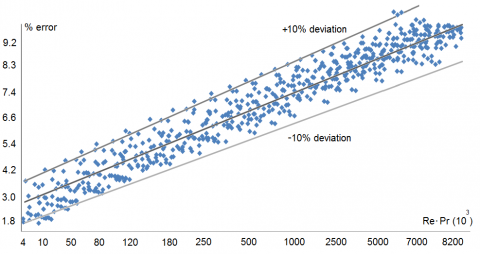Figure 6. Application of the model to experimental data reported by several authors

4. Conclusion

A new model has been obtained for the determination of the heat transfer coefficient in transition and turbulent regime, on a fluid flow in single phase inside straight tubes. The model obtained has a greater range of applicability, covering almost twice the permissible values for the models that were taken as reference, establishing its domain in a range not covered by any model established and known in the literature, for this reason recommends its use in the calculation of the mean coefficients of heat transfer by convection for straight tubes with turbulent flow and transition.

For validity range 2.4∙$10^3$≤Re<$10^4$ and 0.65<Pr≤4.71∙$10^4$, the proposal model correlates with an average error of 13.91%, in 80.32% of the available experimental data. For 2.4∙$10^3$≤Re<$10^4$ and Pr<200, the average error obtained is 6.96% for 90.42% of the available data. For validity range $10^4$≤Re≤8.2∙$10^6$ and 0.65<Pr≤4.71∙$10^4$, the proposal model correlates with an average error of 13.96%, in 80.94% of the available experimental data. For $10^4$≤Re≤8.2∙$10^6$ and Pr<200, the mean error obtained is 7.12 % for 88.35% of the available data.

Acknowledgment

The Doctoral program of the Universidad Central “Marta Abreu” de Las Villas, Santa Clara, Cuba, is gratefully acknowledged.

Nomenclature

a        Thermal diffusivity, m2∙s-1

A       Constant, defined in Equation (74)

B       Constant, defined in Equation (74)

C       Constant, defined in Equation (74)

$C_p$    Fluid specific heat, J∙kg-1∙K-1

$C_W$   Drag coefficient

d        Equivalent inner tube diameter, m

D       Constant, defined in Equation (74)

f        Darcy friction factor

l         Length of the tube, m

L       Initial section of hydrodynamic compensation, m

$L_C$    Mixture length of the energy in the thickness $δ_3$, m

$L_M$   Mixture length of the thickness $δ_2$, m

N        Exponent of the Petukhov correction in Equation (63)

$Nu$     Nusselt number

$Pr$      Prandtl number for single-phase

q*        Total heat flux, kg∙m-2∙s-3

$q_0*$       Heat flux on the boundary layer surface, kg∙m-2∙s-3

$q_{cond}$     Conductive component of the total heat flux, kg∙m-2∙s-3

$q_{turb}$      Turbulent component of the total heat flux, kg∙m-2∙s-3

Re        Reynolds number for single-phase

St        Stanton number

$T_F$       Mean fluid temperature, °C

$T_I$        Instantaneous temperature used in Equation (5), °C

$T_F*$     Temperature fluctuation used in Equation (5), °C

$T_P$      Wall temperature, °C

$V_1$      Velocity at the edge of the viscous layer, m∙s-1

$V_M$     Mean fluid velocity, m∙s-1

$V_X*$     Fluctuation of the $V_X^M$, m∙s-1

$V_Y*$     Fluctuation of the $V_Y^M$, m∙s-1

$V_X^M$    Instantaneous velocity in the coordinate axis x used in Equation (2), m∙s-1

$V_Y^M$    Instantaneous velocity in the coordinate axis y used in Equation (3), m∙s-1

Greek symbols

$α$        Heat transfer coefficient in single-phase, kg∙m-1∙K-1∙s-1

$ε_C$     Heat turbulent diffusivity, m2∙s-1

$ε_M$    Momentum turbulent diffusivity, m2∙s-1

$µ_F$     Fluid dynamic viscosity at TF, kg∙m-1∙s-1

$µ_P$     Fluid dynamic viscosity at TP, kg∙m-1∙s-1

ρ         Fluid density, kg∙m-3

λ         Fluid thermal conductivity, W∙m-1∙K-1

v         Liquid kinematic viscosity, m2∙s-1

$δ_2$      Film thickness of the momentum in boundary layer, m

$δ_3$      Film thickness of the thermal boundary layer, m

τ         Shear stress in the turbulent boundary layer, kg∙m∙s-2

$τ_0$       Shear stress on the surface of the turbulent boundary layer, kg∙m∙s-2

$τ_{Visc}$     Stress of the viscous forces, kg∙m∙s-2

$τ_{Turb}$    Stress of the turbulent strain, kg∙m∙s-2

ΔP         Pressure drop, m

References

 Dirker J, Van der Vyver H. (2004). Convection heat transfer in concentric annuli. Experimental Heat Transfer 17(1): 19-29. http://doi.org/10.1080/08916150490246528

 Liu D, Zheng Y, Moore A, Ferdows M. (2017). Spectral element simulations of three dimensional convective heat transfer. International Journal of Heat and Mass Transfer 111: 1023-1038. http://doi.org/10.1016/j.ijheatmasstransfer.2017.04.066

 Will JB, Kruyt NP, Venner CH. (2017). An experimental study of forced convective heat transfer. International Journal of Heat and Mass Transfer 109: 1059-1067. http://doi.org/10.1016/j.ijheatmasstransfer.2017.02.028

 Cttani L, Bozzoli F, Raineri S. (2017). Experimental study of the transitional flow regime in coiled tubes by the estimation of local convective heat transfer coefficient. International Journal of Heat and Mass Transfer 112: 825-836. http://doi.org/10.1016/j.ijheatmasstransfer.2017.04.066

 Gschnaidtner T, Schatte GA, Kohlhepp A, Wang Y, Wieland C, Spliethoff H. (2018). A new assessment method for the evaluation of supercritical heat transfer correlations, particularly with regard to the “multiple/no solutions” problem. Thermal Science and Engineering Progress 7: 267-278. https://doi.org/10.1016/j.tsep.2018.07.006

 Muhammad-Ali H, Briggs A. (2015). A semi-empirical model for free-convection condensation on horizontal pin-fin tubes. International Journal of Heat and Mass Transfer 81：  157-166. http://dx.doi.org10.1016j.ijheatmasstransfer.2014.10.008

 Zhang W, Du X, Yang L, Yang Y. (2016). Research on performance of finned tube bundles of indirect air-cooled. Mathematical Modelling of Engineering Problems 3(1): 47-51. http://doi.org/10.18280/mmep.030108

 Costa-Magazoni F, Cabezas-Gómez L, Fariñas-Alvariño P, Sáiz-Jabardo JM. (2019). Closed form relationships of temperature effectiveness of cross-flow heat exchangers. Thermal Science and Engineering Progress 9: 110-120. https://doi.org/10.1016/j.tsep.2018.11.005

 Camaraza-Medina Y, Rubio-Gonzales AM, Cruz-Fonticiella OM, García-Morales OF. (2017). Analysis of pressure influence over heat transfer coefficient on air cooled condenser. Journal Européen des Systems Automatisés 50(3): 213-226. http://dx.doi.org/10.3166/jesa.50.213-226

 Elshafei EAM, Awad MM, El-Negiry E, Ali AG. (2010). Heat transfer and pressure drop in corrugated channels. Energy 35(1): 101-110. http://doi.org/10.1016/j.energy.2009.08.031

 Camaraza-Medina Y, Cruz-Fonticiella OM, García- Morales OF. (2018). Predicción de la presión de salida de una turbina acoplada a un condensador de vapor refrigerado por aire. Centro Azúcar 45(1): 50-61.

 Dattas AK, Yanase S, Kochi T, Shatat MME. (2017). Laminar forced convective heat transfer in helical pipe flow. International Journal of Thermal Sciences 120: 41-49. http://doi.org/10.1016/j.ijthermalsci.2017.05.026

 Liu F, Cai Y, Wang L, Zhao J. (2018). Effect of nanoparticle shape on laminar forced convective heat transfer in curved ducts using two-model.  International Journal of Heat and Mass Transfer 116: 825-836. http://doi.org/10.1016/j.ijthermalsci.2017.08.097

 Camaraza-Medina Y, Rubio-Gonzales AM, Cruz-Fonticiella OM, García-Morales OF, Vizcón-Toledo R, Quiza-Sardiñas R. (2018). Simplified analysis of heat transfer through a finned tube bundle in air cooled condenser--second assessment. Mathematical Modelling of Engineering Problems 5(4): 365-372. https://doi.org/10.18280/mmep.050413

 Medina YC, Khandy NH, Fonticiella OMC, Morales OFG. (2017). Abstract of heat transfer coefficient modelation in single-phase systems inside pipes. Mathematical Modelling of Engineering Problems 4(3): 126-131. https://doi.org/10.18280/mmep.040303

 Medina YC, Fonticiella OMC, Morales OFG. (2017). Design and modelation of piping systems by means of use friction factor in the transition turbulent zone. Mathematical Modelling of Engineering Problems 4(4): 162-167. https://doi.org /10.18280/mmep.040404

 Medina YC, Khandy NH, Carlson KM, Fonticiella OMC, Morales OFG. (2018). Mathematical modeling of two-phase media heat transfer coefficient in air cooled condenser system. International Journal of Heat and Technology 36(1): 319-324. https://doi.org/10.18280/ijht.360142

 Camaraza-Medina Y, Hernández-Guerrero A, Luviano-Ortiz JL, Mortensen-Carlson K, Cruz-Fonticiella OM, García-Morales OF. (2019). New model for heat transfer calculation during film condensation inside pipes. International Journal of Heat and Mass Transfer 128: 344-353. https://doi.org/10.1016/j.ijheatmasstransfer.2018.09.012

 Mondal S, Field RW. (2018). Theoretical analysis of the viscosity correction factor for heat transfer in pipe flow. Chemical Engineering Science 187: 27–32. https://doi.org/10.1016/j.ces.2018.04.047

 Camaraza-Medina Y, Khandy NH, Carlson KM, Cruz-Fonticiella OM, García-Morales OF, Reyes-Cabrera D. (2018). Evaluation of condensation heat transfer in air-cooled condenser by dominant flow criteria. Mathematical Modelling of Engineering Problems 5(2): 76-82. https://doi.org /10.18280/mmep.050204

 Binu TV, Jayanti S. (2018). Heat transfer enhancement due to internal circulation within a rising fluid drop. Thermal Science and Engineering Progress 8: 385-396. https://doi.org/10.1016/j.tsep.2018.09.009

 Camaraza Y. (2017). Introducción a la termo transferencia, Editorial Universitaria, La Habana.

 Camaraza-Medina Y, Cruz-Fonticiella OM, Garcia-Morales OF. (2019). New model for heat transfer calculation during fluid flow in single phase inside pipes. Thermal Science and Engineering Progress. https://doi.org/10.1016/j.tsep.2019.03.014